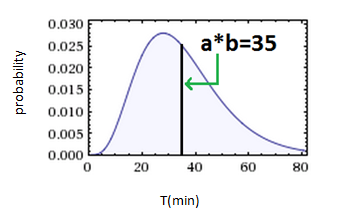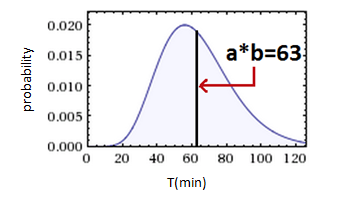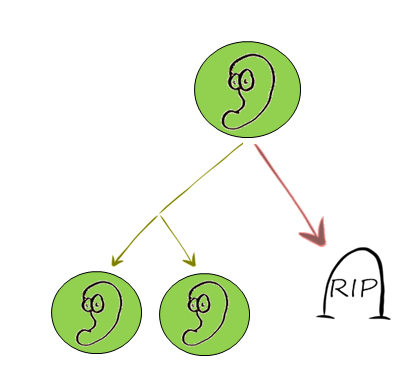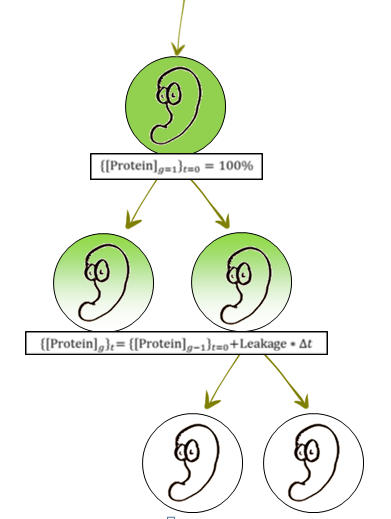# Team:BGU Israel/Model1.html

BGU_Israel

A Stochastic Birth-Death Model

#### Overview

The initial and most important goal of our model was to predict whether a system like ours will work.

In other words, will the system give the bacteria enough time to perform a certain biochemical function, but also ensure that the mechanism won't fail and the bacteria won't survive?

###### The model predicted

1. A possible overall time frame, working time frame, and generation range.
2. Limits of leakage rates and mechanism strengths parameters the system can handle.

At first, we aimed to construct a deterministic model that describes the population's behavior based on a set of differential equations.
We very quickly realized that a reliable deterministic model will require precise, deterministic parameters for the commonly used building blocks of SynBio.

We found out that the variation of these values can be enormous- what is the copy number of each plasmid and does it remain constant? What are the transcription and translation rates for each gene? What are the degradation rates for the different components? What is the promoter’s strength and what is its basal expression? And on top of all those complications, even if one can obtain a good estimation of the quantitative data, will it remain the same when integrated in a novel system with new interconnections and dependencies?

For example, it has been shown that gene expression can vary in response to changing the reporter gene alone by up to 44% in a simple expression circuit .

We decided that a factor of uncertainty had to be incorporated into the model, and therefore, we decided to construct a stochastic birth-death model that will model the behavior of the entire bacterial population, based on the probability of each and every individual to die or to proliferate.

###### How does it work?

In both P.A.S.E 1 and P.A.S.E 2 a common critical element is that a protein is produced while the bacterium is in the lab, keeping the cell alive, and stops being produced when it is released from the lab:

1. In P.A.S.E 1, a protective protein- the CI repressor, represses the production of the Holin & Endolysin toxins.
2. In P.A.S.E 2, an Essential protein- tyrosine tRNA synthetase, a protein that the cell requires to function.

This protein concentration directly affects the probability of each individual bacterium to die or to proliferate.

###### So what are the mathematics behind the model?

There is evidence  that the distributions for cell division times and death times are similar to the Gamma distribution.

In the time frame [0,t], 0 is the moment the cell has finished its last division, and ‘t’ is either the time of death of the cell, or its proliferation time.

What’s important to know about the Gamma Probability Density Function?

The Gamma(a,b) PDF is defined by its parameters-
‘a’ is shape (dimensionless) , ‘b’ is scale (minutes in our case). The mean of the distribution equals ‘a*b’ and represents the average interdivision time or average death time. Controlling the probabilities is controlling these parameters!

#### In lab

1. Right after an individual cell has divided (t=0), in order for a second proliferation to happen, a set of events in the cell cycle need to occur. Therefore, proliferation probability is low right after the division, rises as time advances, and falls again asymmetrically as time goes by after average inter-division time.2. Similar to proliferation, Death time is deeply dependent on critical steps that can fail in the cell cycle, thus giving it a similar shape function.###### Initialization

In the lab, when the production of critical proteins in P.A.S.E 1 and 2 is fully induced, protein concentration remains constantly 100% of its possible concentration. This is the initialization state, and in this state each option, dying or proliferating, was assigned a unique time-dependent gamma PDF (Probability Density Function). The PDF’s assigned were such that a higher probability is given to cell proliferation (assuming infinite resources in the lab), and produced a typical bacterial growth curve with generation time of 40 minutes- similar to that of engineered E. coli.

#### In vivo

3. Bacteria is released and is no longer induced. The first generation (g=1) has 100% protein concentration, and it begins to drop.
The algorithm (which is based on Gillespie’s algorithm) draws death and proliferation times for each individual, and makes a decision if the cell will divide or not.

4. The probabilities to die or to proliferate for each individual are dependent on the protein concentration. As explained above, controlling the probabilities controls the shape (‘a’) and scale (‘b’) parameters, so ‘a’ of death gamma PDF is changed according to the following equation:
A=slope*[protein]+n
As protein conc. drops, the death PDF gets a positive skew and death of bacteria becomes more preferable, once it is low enough, population begins to die out.

5. It is known that every gene expression mechanism will have some sort of leakage. Because leakage is difficult to predict before experimental testing, we modeled a range of possible leakages. What began as risk factor for our system (high leakage means our system will not die out), generated an idea for modularity-
By creating a library of different promoters with different leakages generated from deliberated mutations in the original promoter, we can achieve a programmable system!

6. Mechanism strength, the second parameter we modeled, was simply introduced by changing the ‘slope’ and ‘n’ parameters to represent a stronger influence of [protein] on ‘a’ (the shape parameter).
It represents a series of factors of how the protein interacts with life\death related mechanisms in the cell.
A stronger mechanism means a faster decline of 'a' (shape parameter) as [protein] decreases.25 Combinations of different Leakage rates and mechanism strengths were simulated in-silico with 30 repeats for each combination.